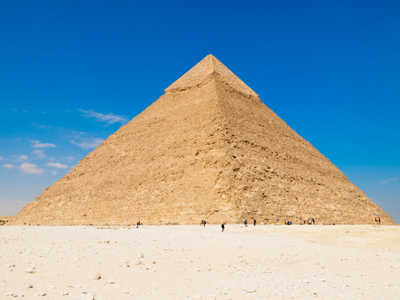A pyramid has a square face and four triangular faces.

# Shapes 1

This Math quiz is called 'Shapes 1' and it has been written by teachers to help you if you are studying the subject at middle school. Playing educational quizzes is a fabulous way to learn if you are in the 6th, 7th or 8th grade - aged 11 to 14.

It costs only \$12.50 per month to play this quiz and over 3,500 others that help you with your school work. You can subscribe on the page at Join Us

Shapes have angles, faces and edges. Shapes can be two-dimensional (2D) or three-dimensional (3D). 2D shapes are flat, like triangles and squares and 3D shapes are solid, like cubes and pyramids.There are many different shapes. Do you have a favorite?

How much do you know about the different shapes? Can you tell the difference between squares and cubes? Do you know what vertices are? Find out by playing this quiz all about shapes.

1.
Which shape is half of a circle?
Semicircle
Hemisphere
Oval
Prism
A hemisphere is half of a sphere
2.
What is the name for all polygons with 4 sides?
Square
Cube
Rectangle
A square and a rectangle are both quadrilaterals
3.
How many sides does a hexagon have?
5
6
7
8
One way to remember this is that both heXagon and siX have a letter X in them
4.
How many faces does a cube have?
2
4
6
8
Every face is a square
5.
What is the name of the shape with five sides?
Pentagon
Hexagon
Octagon
Cylinder
Pentagons have 5 sides and a pentagram is a 5 pointed star
6.
What are the corners of a shape also known as?
Faces
Angles
Edges
Vertices
The singular term for vertices is vertex
7.
What is the name of the shape that resembles a ball?
Hemisphere
Cone
Sphere
Semicircle
A sphere is like a 3 dimensional circle
8.
Which shape has only one edge?
Square
Circle
Triangle
Pentagon
A circle has no vertices
9.
Which 3D shape has three faces and two edges?
Cube
Cylinder
Prism
Cone
A cylinder is like a tube with circles at each end
10.
Which shape has a square face and four triangular faces?
Prism
Cylinder
Pyramid
Sphere
The pyramids in Egypt are ancient buildings which are the shape of a pyramid
Author:  Amanda Swift